Updating search results...

# 52 Results

View
Selected filters:
• NC.Math.8.EE.7Rating
0.0 stars

Students will review the definition of 'like terms' and combining like terms. They will then learn to apply this to adding polynomials. Students will have an opportunity to work practice problems as they are viewing a PowerPoint presentation of the lesson. They will be adding polynomials using both the horizontal method and the vertical method.

Subject:
Mathematics
Material Type:
Lesson Plan
Provider:
Alabama Learning Exchange
Author:
Sheila Mitchell
02/26/2019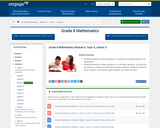Conditional Remix & Share Permitted
CC BY-NC-SA
Rating
0.0 stars

In this lesson, students know that a linear equation is a statement of equality between two expressions. Students know that a linear equation in x is actually a question: Can you find all numbers x, if they exist, that satisfy a given equation? Students know that those numbers x that satisfy a given equation are called solutions.

Subject:
Mathematics
Material Type:
Lesson Plan
Provider:
EngageNY
02/17/2017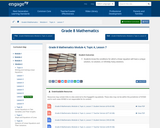Conditional Remix & Share Permitted
CC BY-NC-SA
Rating
0.0 stars

In this lesson, students know the conditions for which a linear equation will have a unique solution, no solution, or infinitely many solutions.

Subject:
Mathematics
Material Type:
Lesson Plan
Provider:
EngageNY
02/17/2017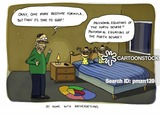Unrestricted Use
CC BY
Rating
0.0 stars

This resource is designed to assist students understanding of real-world applicaitono f mathematical concepts.&nbsp; Students will complete their investigation over the course of 4-5 days, following the multi-segment program &quot;Mathnet&quot; from the PBS series &quot;Square One Television&quot;&nbsp;While watching the day&#39;s segment, students will be recording in their notebooks the following information: math concepts used/referred to, important clues about the investigation, and individuals who they believe are &quot;persons of interest.&quot;&nbsp; Following the last video segmnet, students will complete their I&quot;Investigation Report&quot; for submission to their supervisor (a.k.a the teacher).

Subject:
Algebra
Geometry
Math 1
Material Type:
Activity/Lab
Author:
Nathan Rutko
07/02/2020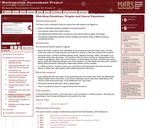Only Sharing Permitted
CC BY-NC-ND
Rating
0.0 stars

This lesson unit is intended to help you assess how well students use algebra in context, and in particular, how well students: explore relationships between variables in everyday situations; find unknown values from known values; find relationships between pairs of unknowns, and express these as tables and graphs; and find general relationships between several variables, and express these in different ways by rearranging formulae.

Subject:
Algebra
Mathematics
Material Type:
Assessment
Lesson Plan
Provider:
Shell Center for Mathematical Education
Provider Set:
Mathematics Assessment Project (MAP)
07/31/2019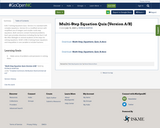Conditional Remix & Share Permitted
CC BY-NC
Rating
0.0 stars

8.EE.7 Solving Equations Quiz. Version A is standard with complex equations. Version B is a modified version with simplified use of integers and smaller multi-step equations. Both versions contain fractional problems. Each quiz provides directions including the tip Don't Call Me After Midnight to remind students of the steps for solving equations.

NCM1.A-REI.3 Solving linear equations and inequalities in one variable to include fractions.

Subject:
Algebra
Mathematics
Material Type:
Assessment
07/10/2020Conditional Remix & Share Permitted
CC BY-NC
Rating
0.0 stars

8.EE.7 Solving Equations Quiz. Two versions. M is the standard version M1 is the modified version with simplified negatives and smaller multi-step equations. Both versions contain fractional problems too.

Subject:
Algebra
Mathematics
Material Type:
Assessment
11/06/2019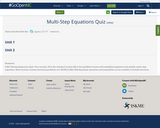Conditional Remix & Share Permitted
CC BY-NC
Rating
0.0 stars

8.EE.7 Solving Equations Quiz. Two versions. M is the standard version M1 is the modified version with simplified negatives and smaller multi-step equations. Both versions contain fractional problems too.

NCM1.A-REI.3 Solving linear equations and inequalities in one variable to include fractions.

Subject:
Algebra
Geometry
Mathematics
Material Type:
Assessment
06/26/2020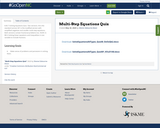Conditional Remix & Share Permitted
CC BY-NC
Rating
0.0 stars

8.EE.7 Solving Equations Quiz. Two versions. M is the standard version M1 is the modified version with simplified negatives and smaller multi-step equations. Both versions contain fractional problems too.

NCM1.A-REI.3 Solving linear equations and inequalities in one variable to include fractions.

Subject:
Algebra
Mathematics
Material Type:
Assessment
05/30/2020Rating
0.0 stars

The following materials include a Common Core-aligned task and formative assessments which may be integrated into a currently existing curriculum. The final task assesses student mastery of the standards related to linear functions.

Subject:
Mathematics
Material Type:
Activity/Lab
Assessment
Unit of Study
Provider:
The New York City Department of Education
Author:
The New York City Department of Education
02/26/2019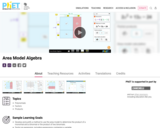Unrestricted Use
CC BY
Rating
0.0 stars

Develop and justify a method to use the area model to determine the product of a monomial and a binomial or the product of two binomials.
Factor an expression, including expressions containing a variable.
Recognize that area represents the product of two numbers and is additive.
Represent a multiplication problem as the area of a rectangle, proportionally or using generic area.
Develop and justify a strategy to determine the product of two multi-digit numbers by representing the product as an area or the sum of areas.

Subject:
Mathematics
Material Type:
Activity/Lab
Author:
PhET Interactive Simulations
10/01/2021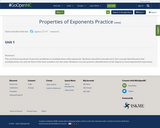Conditional Remix & Share Permitted
CC BY-NC-SA
Rating
0.0 stars

The worksheet presents 14 practice problems in multiplication with exponents. Students should be introduced to the concept identification that multiplication can only be done if the base numbers are the same. Students can also practice identification of the degree in each exponential expression.

Subject:
Math 1
Mathematics
Material Type:
Homework/Assignment
Presentation
Teaching/Learning Strategy
06/26/2020Conditional Remix & Share Permitted
CC BY-NC
Rating
0.0 stars

8.EE.7 Solving Equations Quiz. Two versions. M is the standard version M1 is the modified version with simplified negatives and smaller multi-step equations. Both versions contain fractional problems too.

NCM1.A-REI.3 Solving linear equations and inequalities in one variable to include fractions.

Subject:
Algebra
Geometry
Mathematics
Material Type:
Assessment
07/15/2020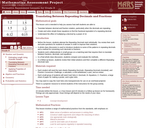Only Sharing Permitted
CC BY-NC-ND
Rating
0.0 stars

This lesson unit is intended to help teachers assess how well students are able to: translate between decimal and fraction notation, particularly when the decimals are repeating; create and solve simple linear equations to find the fractional equivalent of a repeating decimal; and understand the effect of multiplying a decimal by a power of 10.

Subject:
Algebra
Geometry
Mathematics
Material Type:
Assessment
Lesson Plan
Provider:
Shell Center for Mathematical Education
Provider Set:
Mathematics Assessment Project (MAP)
06/24/2019Conditional Remix & Share Permitted
CC BY-NC-SA
Rating
0.0 stars

This course was created by the Rethink Education Content Development Team in partnership with the North Carolina Virtual Public Schools. This course is aligned to the NC Standards for 8th Grade Math.&nbsp;

Subject:
Mathematics
Material Type:
Activity/Lab
Assessment
Formative Assessment
Full Course
Homework/Assignment
Lecture Notes
Vocabulary
Author:
AMBER GARVEY
08/29/2022Conditional Remix & Share Permitted
CC BY-NC-SA
Rating
0.0 stars

This course was created by the Rethink Education Content Development Team in partnership with the North Carolina Virtual Public Schools. This course is aligned to the NC Standards for 8th Grade Math.&nbsp;

Subject:
Mathematics
Material Type:
Full Course
Author:
AMBER GARVEY
02/01/2022Conditional Remix & Share Permitted
CC BY-NC-SA
Rating
0.0 stars

This course was created by the Rethink Education Content Development Team in partnership with the North Carolina Virtual Public Schools. This course is aligned to the NC Standards for 8th Grade Math.&nbsp;

Subject:
Mathematics
Material Type:
Activity/Lab
Assessment
Formative Assessment
Full Course
Lesson
Module
Vocabulary
Author:
AMBER GARVEY
03/03/2022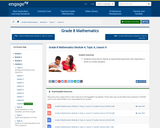Conditional Remix & Share Permitted
CC BY-NC-SA
Rating
0.0 stars

In this lesson, students know how to rewrite an exponential expression that represents a series as a linear equation.

Subject:
Mathematics
Material Type:
Lesson Plan
Provider:
EngageNY
02/17/2017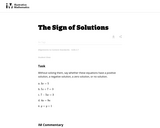Unrestricted Use
CC BY
Rating
0.0 stars

It is possible to say a lot about the solution to an equation without actually solving it, just by looking at the structure and operations that make up the equation. This exercise turns the focus away from the familiar Ňfinding the solutionÓ problem to thinking about what it really means for a number to be a solution of an equation.

Subject:
Mathematics
Material Type:
Activity/Lab
Provider:
Illustrative Mathematics
Provider Set:
Illustrative Mathematics
Author:
Illustrative Mathematics
05/01/2012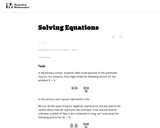Unrestricted Use
CC BY
Rating
0.0 stars

This task requires students to think about equations and solve them using pictures.

Subject:
Mathematics
Material Type:
Activity/Lab
Provider:
Illustrative Mathematics
Provider Set:
Illustrative Mathematics
Author:
Illustrative Mathematics